Question 1: Find the mean of the following set of numbers:
(i) 6, 9, 11, 12 and 7
(ii) 11, 14, 23, 26, 10, 12, 18 and 6

Solution 1:
(i) x̄ = (x1 + x2 +...+ xn)/5
Here n = 5
∴ x̄ = (6.9 + 11 + 12 + 7)/5 = 45/5 = 9

(ii) x̄ = (x1  + x2 +…+ xn)/n
Here  n = 8
∴ x̄ = (11 + 14 + 23 + 26 + 10 + 12 + 18 + 6)/8 = 120/8 = 15

Question 2: Marks obtained in mathematics) by 9 students are given below:
60, 67, 52, 76, 50, 51, 74, 45 and 56
(a) find the arithmetic mean
(b) if marks of each student be increased by 4; what will be the new value of arithmetic mean.

Solution 2: (a) Here n = 9
x̄ = (x1 + x2 +……+ xn)/n
∴ x̄ = (60 + 67 + 52 + 76 + 50 + 51 + 74 + 45 + 56)/9 = 531/9 = 59

(b) If marks of each student be increased by 4 then new arithmetic mean will be = 59 + 4 = 63

Question 3: Find the mean of the natural numbers from 3 to 12.

Solution 3: Numbers between 3 to 12 are 3, 4, 5, 6, 7, 8, 9, 10, 11 and 12.
Here n = 10
x̄ = (x1 + x2 +…….xn)/n
∴ x̄ = (3 + 4 + 5 + 6 + 7 + 8 + 9 + 10 + 11 + 12)/10 = 75/10 = 7.5

Question 4:
(a) Find the mean of 7, 11, 6, 5, and 6
(b) If each number given in (a) is diminished by 2, find the new value of the mean.

Solution 4: (a) The mean of 7, 11, 6, 5 and 6
x̄ = (7 + 11 + 6 + 5 + 6)/5 = 35/5 = 7

(b) If we subtract 2 from each number, then the mean will be 7-2 = 5

Question 5: The mean of the following frequency distribution is 21 1/7. Find the value of ‘f’.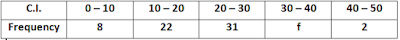Solution 5: x̄ = 21 1/7 = 148/7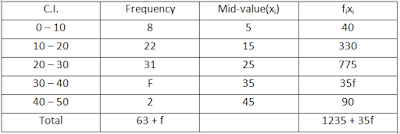x̄ = Σfixi/Σfi = (1235 + 35f)/(63 + f)
⇒ 148 = (1235 + 35f)/(63 + f)
⇒ 9324 + 148f = 8645 + 245f
⇒ 245f – 148f = 9324 – 8645
⇒ f = 679/97
⇒ f = 7

Question 6: Using step-deviation method, calculate the mean marks of the following distribution.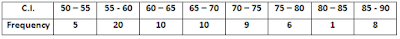Solution 6:
Let the assumed mean A = 72.5Mean = A + Σfidi/Σfi = 72.5 + (-280/80) = 69

Question 7: Using the information given in the adjoining histogram, calculate the mean.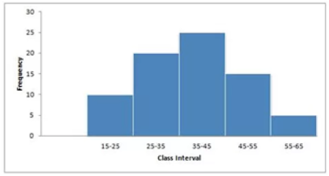Solution 7: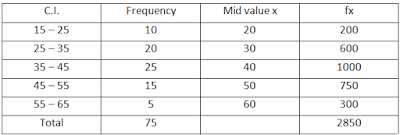x̄ = Σfx/Σf = 2850/75 = 38

Question 8: By drawing an ogive, estimate the median for the following frequency distribution: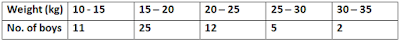Solution 8:Number of terms = 55
∴ Median = (55 + 1th)/2 term = 28th termThrough mark of 28 on the y-axis, draw a line parallel to x-axis which meets the curve at A, From A, draw a perpendicular to x-axis, which meets x-axis at B.
The value of B is the median which is 18.4 kg

Question 9: The marks obtained by 120 students in a mathematics test is given below:Draw an ogive for the given distribution on a graph sheet. Use a suitable scale for your ogive. Use your ogive to estimate:
(i) the median
(ii) the number of students who obtained more than 75% in test.
(iii) the number of students who did not pass in the test if the pass percentage was 40.
(iv) the lower quartile

Solution 9:(i) Median  = (120 + 1)/2 = 60.5th  term
Through mark 60.5, draw a parallel line to x-axis which meets the curve at A. From A draw a perpendicular to x-axis meeting it at B.
The value of point B is the median = 43

(ii) Number of students who obtained up to 75% marks in the test = 120
Number of students who obtained more than 75% mark in the test = 120 – 110 = 10

(iii) Number of students who obtained less than 40% marks in the test = 52(from the graph;x = 40, y = 52)

(iv) Lower quartile = Q1 = 120 × ¼ = 30th term = 30

Question 10: Find the mean for the following frequency distribution:Solution 10:Mean = Σfx/Σf = 7150/50 = 143